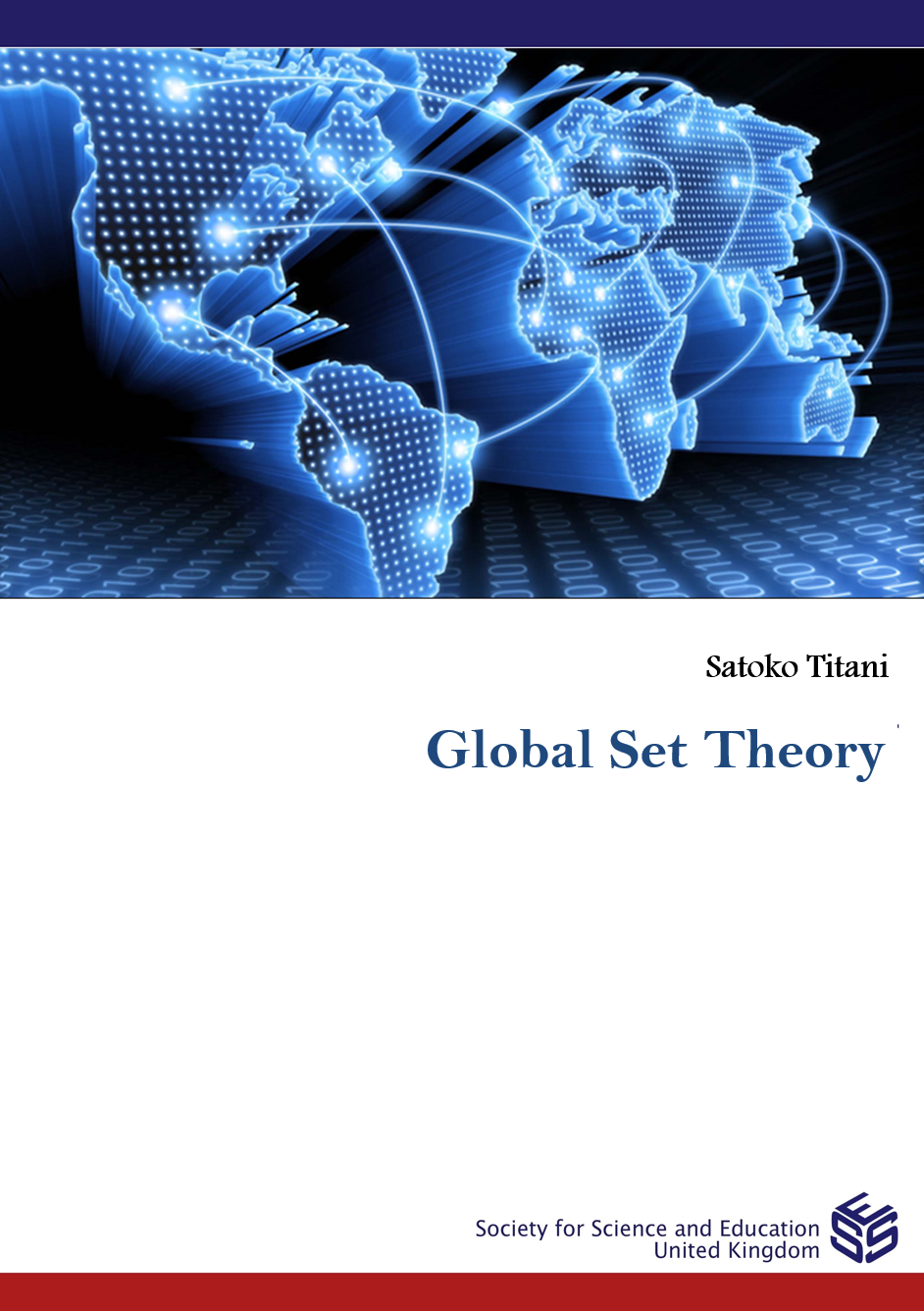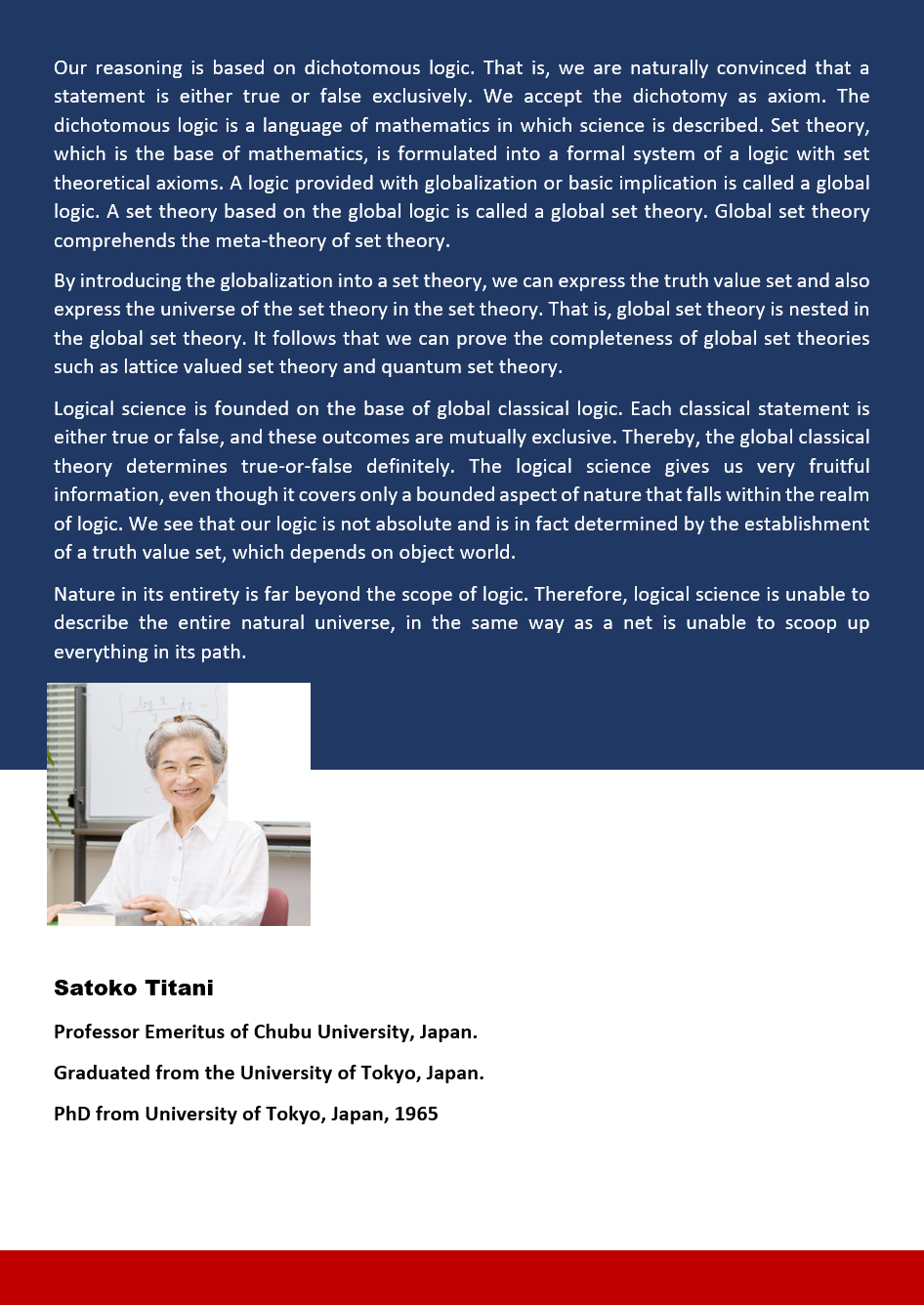# Global Set TheoryGlobal Set Theory

Satoko Titani

Professor Emeritus of Chubu University, Japan.

Our reasoning is based on dichotomous logic. That is, we are naturally convinced that a statement is either true or false exclusively. We accept the dichotomy as axiom. The dichotomous logic is a language of mathematics in which science is described. Set theory, which is the base of mathematics, is formulated into a formal system of a logic with set theoretical axioms. A logic provided with globalization or basic implication is called a global logic. A set theory based on the global logic is called a global set theory. Global set theory comprehends the meta-theory of set theory.
By introducing the globalization into a set theory, we can express the truth value set and also express the universe of the set theory in the set theory. That is, global set theory is nested in the global set theory. It follows that we can prove the completeness of global set theories such as lattice valued set theory and quantum set theory.
Logical science is founded on the base of global classical logic. Each classical statement is either true or false, and these outcomes are mutually exclusive. Thereby, the global classical theory determines true-or-false definitely. The logical science gives us very fruitful information, even though it covers only a bounded aspect of nature that falls within the realm of logic. We see that our logic is not absolute and is in fact determined by the establishment of a truth value set, which depends on object world.
Nature in its entirety is far beyond the scope of logic. Therefore, logical science is unable to describe the entire natural universe, in the same way as a net is unable to scoop up everything in its path.

DOI: 10.14738/tnc.092018.1